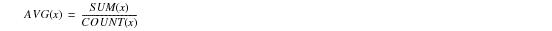# 15.00 - Using Aggregate Join Indexes - Teradata Database

prodname
vrm_release
15.00
category
User Guide
featnum
B035-1094-015K

### Using Aggregate Join Indexes

Aggregate join indexes offer an extremely efficient, cost-effective method of resolving requests that frequently specify the same aggregation operations on the same column or columns. When aggregate join indexes are available, the system does not have to repeat aggregation calculations for every request.

You can define an aggregate join index on two or more tables or on a single table. A single‑table aggregate join index includes:

• A subset of the columns in a base table
• Additional columns for the aggregate summaries of the base-table columns
• You can create an aggregate join index using the GROUP BY clause and the following built‑in aggregate functions.

• SUM
• COUNT
• MAX
• MIN
• The following restrictions apply to defining an aggregate join index.

• Only the COUNT, MAX, MIN, and SUM aggregate functions are valid in any combination.
• COUNT DISTINCT and SUM DISTINCT are not valid.

• To avoid overflow, always type the COUNT, MAX, MIN, and SUM columns in an aggregate join index definition as FLOAT.
• Teradata Database enforces this restriction as follows.

 IF you … THEN Teradata Database … do not define an explicit data type for a COUNT, MAX, MIN, or SUM column assigns the FLOAT data type to it automatically. define a COUNT, MAX, MIN, or SUM column as anything other than FLOAT returns an error and does not create the aggregate join index.

Many aggregate functions are based on the SUM, MAX, MIN, and COUNT functions, so even though you cannot specify many individual aggregate functions in an aggregate join index, you can combine these 4 functions in a number of ways to create an aggregate join index to resolve requests that use more complicated aggregate functions.

A simple example is using the COUNT and SUM functions to compute an average.